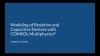# Learning Center Resources

Course:

Modeling Resistive and Capacitive Devices

## Modeling Capacitance Matrices

In Part 4 of our course providing an introduction to modeling resistive and capacitive devices, we continue with discussing the modeling of capacitive systems, wherein there are multiple electrodes. We begin by talking about the Stationary Source Sweep study and explaining what the study is, what the study does, and how to utilize it when building a model with multiple electrodes. We demonstrate its use first with a 2D example and then a 3D example. We then review some alternative strategies that can be used with the Stationary Source Sweep study type that should be considered when computing models with a large number of terminals.

From there, we return to the model we originally started with in the session and show how to postprocess the results to obtain a quality visualization of all of the fields around the electrode, including displaying the electric field, electric potential, and electric energy density. We then modify the model to introduce a dielectric, and after computing the model, see how the dielectric perturbs the fields. We also show another way to evaluate the terminal capacitance. Thereafter, we discuss the different approaches that can be used to move the dielectric around in the model, such as through a parametric sweep, auxiliary sweep, and the Moving Mesh functionality. Upon computing the model, we then show how to set up a 1D plot to visualize the variation of the capacitance as the dielectric moves. Going further, we show how to perform a sensitivity analysis, using the Sensitivity interface and Sensitivity study step, to determine the derivative of the curve in the plot.

Lastly, we conclude by showing how to model capacitance matrices in both 2D and then 3D using the boundary element method.